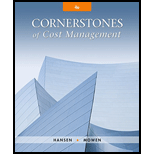# Friedman Company is considering installing a new IT system. The cost of the new system is estimated to be $2,250,000, but it would produce after-tax savings of$450,000 per year in labor costs. The estimated life of the new system is 10 years, with no salvage value expected. Intrigued by the possibility of saving $450,000 per year and having a more reliable information system, the president of Friedman has asked for an analysis of the project’s economic viability. All capital projects are required to earn at least the firm’s cost of capital, which is 12 percent. Required: 1. Calculate the project’s internal rate of return. Should the company acquire the new IT system? 2. Suppose that savings are less than claimed. Calculate the minimum annual cash savings that must be realized for the project to earn a rate equal to the firm’s cost of capital. Comment on the safety margin that exists, if any. 3. Suppose that the life of the IT system is overestimated by two years. Repeat Requirements 1 and 2 under this assumption. Comment on the usefulness of this information.BuyFindarrow_forward ### Cornerstones of Cost Management (C... 4th Edition Don R. Hansen + 1 other Publisher: Cengage Learning ISBN: 9781305970663 #### Solutions Chapter SectionBuyFindarrow_forward ### Cornerstones of Cost Management (C... 4th Edition Don R. Hansen + 1 other Publisher: Cengage Learning ISBN: 9781305970663 Chapter 19, Problem 28P Textbook Problem 47 views ## Friedman Company is considering installing a new IT system. The cost of the new system is estimated to be$2,250,000, but it would produce after-tax savings of $450,000 per year in labor costs. The estimated life of the new system is 10 years, with no salvage value expected. Intrigued by the possibility of saving$450,000 per year and having a more reliable information system, the president of Friedman has asked for an analysis of the project’s economic viability. All capital projects are required to earn at least the firm’s cost of capital, which is 12 percent.Required: 1. Calculate the project’s internal rate of return. Should the company acquire the new IT system? 2. Suppose that savings are less than claimed. Calculate the minimum annual cash savings that must be realized for the project to earn a rate equal to the firm’s cost of capital. Comment on the safety margin that exists, if any. 3. Suppose that the life of the IT system is overestimated by two years. Repeat Requirements 1 and 2 under this assumption. Comment on the usefulness of this information.

1.

To determine

Ascertain the internal rate of return of the given project and describe whether the company should acquire the new IT system.

### Explanation of Solution

Internal rate of return method (IRR): Internal rate of return method is one of the capital investment methods which determine the rate of return, wherein the net present value of all the cash flows (both positive and negative) from an investment is zero. This method is also called as the time-adjusted rate of return method. It used to evaluate the different proposal’s expected rate of return.

Internal rate of return (IRR):

When the annual cash flows are equal, the internal rate of return can determined as follows:

Present value factor for an annuity of $1}=Amount investedEqual annual net cash flows=$2,250,000\$450,000=5

2.

To determine

Ascertain the minimum annual cash flow that must be realized for the given project and interpret on the safety margin that exists.

3.

To determine

Ascertain the internal rate of return and the minimum annual cash flow of the given project, assume that the life of the IT system is overestimated by two years.

### Still sussing out bartleby?

Check out a sample textbook solution.

See a sample solution

#### The Solution to Your Study Problems

Bartleby provides explanations to thousands of textbook problems written by our experts, many with advanced degrees!

Get Started